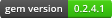# histogram

Generates histograms similar to R's hist and numpy's histogram functions. Inspired somewhat by Richard Cottons's matlab implementation and the wikipedia histogram article.

Tested on latest MRI 1.9.x, 2.x, and jruby (no NArray support for jruby). Known to work on MRI 1.8.7.

### Typical usage:

``````require 'histogram/array'  # enables Array#histogram

data = [0,1,2,2,2,2,2,3,3,3,3,3,3,3,3,3,5,5,9,9,10]
# by default, uses Scott's method to calculate optimal number of bins
# and the bin values are midpoints between the bin edges
(bins, freqs) = data.histogram
# equivalent to:  data.histogram(:scott, :bin_boundary => :avg)
``````

### Multiple types of binning behavior:

``````# :scott, :fd, :sturges, or :middle
data.histogram(:fd)  # use Freedman-Diaconis method to calc num bins
data.histogram(:middle) # (median value between the three methods)
(bins, freqs) = data.histogram(20)                         # use 20 bins
(bins, freqs) = data.histogram([-3,-1,4,5,6])              # custom bins

(bins, freqs) = data.histogram(10, :min => 2, :max => 12)  # 10 bins with set min and max

# bins are midpoints, but can be set as minima
(bins, freqs) = data.histogram([-3,-1,4,5,6], :bin_boundary => :min) # custom bins with :min

# can also set the bin_width (which interpolates between the min and max of the set)
(bins, freqs) = data.histogram(:bin_width => 0.5)
``````

### Multiple Datasets:

Sometimes, we want to create histograms where the bins are calculated based on all the data sets. That way, the resulting frequencies will all line up:

``````# returns [bins, freq1, freq2 ...]
(bins, *freqs) = set1.histogram(30, :other_sets => [[3,3,4,4,5], [-1,0,0,3,3,6]])
``````

### Histograms with weights/fractions:

``````# histogramming with weights
data.histogram(20, :weights => [3,3,8,8,9,9,3,3,3,3])
``````

### Works with NArray objects

``````require 'histogram/narray'    # enables NArray#histogram
# if the calling object is an NArray, the output is two NArrays:
(bins, freqs) = NArray.float(20).random!(3).histogram(20)
# bins and freqs are both NArray.float objects
``````

## Installation

``````gem install histogram
``````

## Acknowledgements

Big thanks to those who have made contributions!